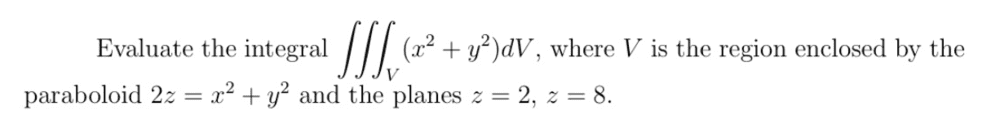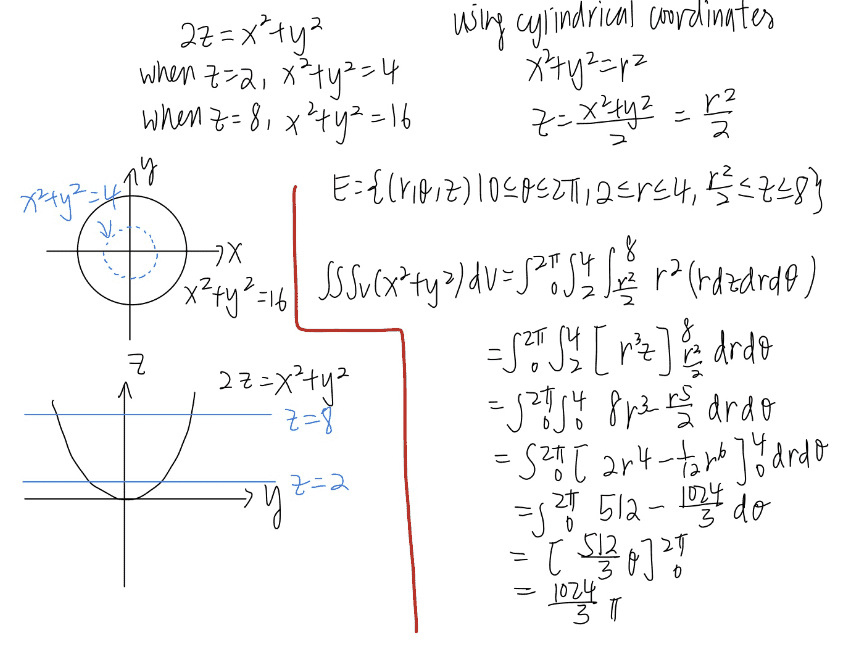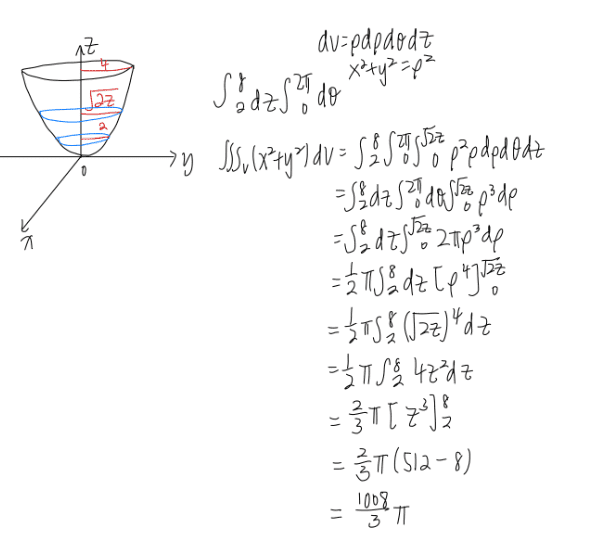# Solving this triple integral

daphnelee-mh
Homework Statement:
(question is attached below)
Relevant Equations:
x^2+y^2=r^2
dV=rdrdzdΘI am trying to solve it using cylindrical coordinates, but I am not sure whether the my description of region E is correct, whether is the value of r is 2 to 4, or have to evaluate the volume 2 times ( r from 0 to 4 minus r from 0 to 2), and whether is okay to take z from r^2/2 to 8

Gold Member
$$\int_2^8 dz \int_0^{2z} 2\pi \rho^3 d\rho$$

•Delta2
daphnelee-mh
May I know which method did you use? I have no idea of it, thanks

Homework Helper
Gold Member
$$\int_2^8 dz \int_0^{2z} 2\pi \rho^3 d\rho$$
Shouldn't the boundary of integration be ##\sqrt{2z}## instead of ##2z##?

•anuttarasammyak
Gold Member
Yes. Thanks for correction.

•Delta2
daphnelee-mhCan you help to see whether this is correct ? Thank you

Homework Helper
Gold Member
Yes it looks correct to me.

daphnelee-mh
Thank you so much

Homework Helper
Gold Member
2022 Award
View attachment 265726
Can you help to see whether this is correct ? Thank you
The digits of 1008 add to 9, so...

daphnelee-mh
The digits of 1008 add to 9, so...
?